# TN Class 8th Maths Solutions Chapter 1 Numbers Ex 1.5

## TN Class 8 Maths Solutions Chapter 1 Numbers Ex 1.5

Question 1.
Fill in the blanks
(i) The ones digits in the cube of 73 is __________ .
7

(ii) The maximum number of digits in the cube of a two digit number is __________ .
6(iii) The smallest number to be added to 3333 to make it a perfect cube is __________ .
42

(iv) The cube root of 540×50 is __________ .
30

(v) The cube root of 0.000004913 is __________ .
0.017

Question 2.
Say True or False.
(i) The cube of 24 ends with the digit 4.
True

(ii) Subtracting 103 from 1729 gives 93.
True(iii) The cube of 0.0012 is 0.000001728.
False

(iv) 79570 is not a perfect cube.
True

(v) The cube root of 250047 is 63.
True

Question 3.
Show that 1944 is not a perfect cube.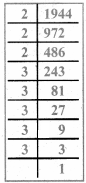1944 = 2 × 2 × 2 × 3 × 3 × 3 × 3 × 3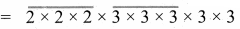= 23 × 33 × 3 × 3
There are two triplets to make further triplets we need one more 3.
∴ 1944 is not a perfect cube.Question 4.
Find the smallest number by which 10985 should be divided so that the quotient is a perfect cube.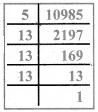We have 10985 = 5 × 13 ×13 × 13
= 5 × 13 ×13 × 13
Here we have a triplet of 13 and we are left over with 5.
If we divide 10985 by 5, the new number will be a perfect cube.
∴ The required number is 5.

Question 5.
Find the smallest number by which 200 should be multiplied to make it a perfect cube.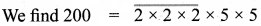Grouping the prime factors of 200 as triplets, we are left with 5 × 5
We need one more 5 to make it a perfect cube.
So to make 200 a perfect cube multiply both sides by 5.1000 = 2 × 2 × 2 × 5 × 5 × 5 × 5 × 5
Now 1000 is a perfect cube.
∴ The required number is 5.Question 6.
Find the cube root 24 × 36 × 80 × 25.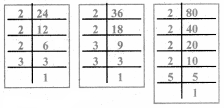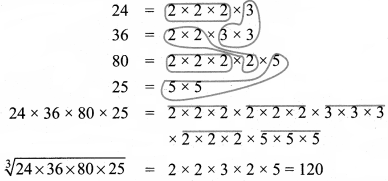Question 7.
Find the cube root of 729 and 6859 prime factorisation.
(i)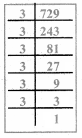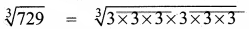= 3 × 3
$$\sqrt{729}$$ = 9(ii) $$\sqrt{6859}$$ = $$\sqrt{19 \times 19 \times 19}$$
$$\sqrt{6859}$$ = 19

Question 8.
What is the square root of cube root of 46656?We have to find out $$\sqrt{(\sqrt{46656})}$$
First we will find $$\sqrt{46656}$$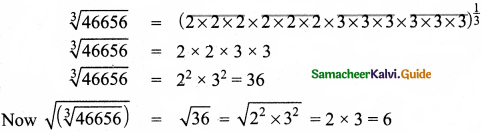∴ The required number is 6.Question 9.
If the cube of a squared number is 729, find the square root of that number.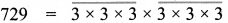(729)1/3 = 3 × 3 = 9
∴ The cube of 9 is 729.
9 = 3 × 3 [ie 3 is squared to get 9]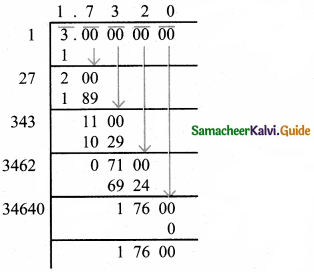We have to find out √3,
√3 = 1.732Question 10.
Find two smallest perfect square numbers which when multiplied together gives a perfect cube number.